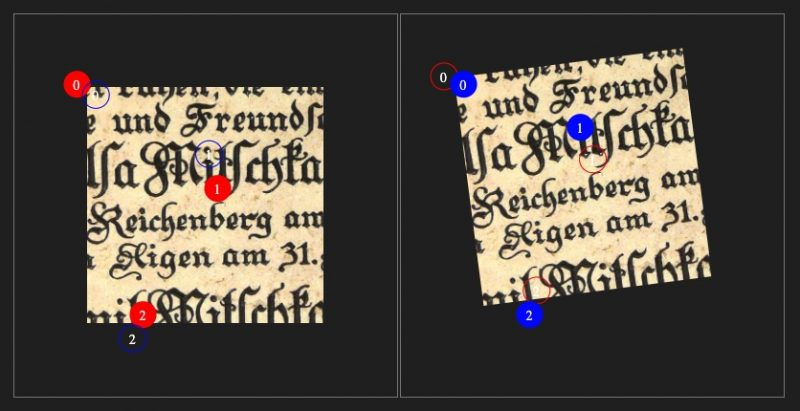# Nudged: a fast transformation estimatorAs a part of my master’s thesis and my work at Infant Cognition Laboratory, I developed an open source JavaScript and Python software package called nudged. It is an easy tool to solve the following optimization problem that is often faced when a real-world object, like a hand or head moves and we want to know how it moved.

As demonstrated in the picture above, we have a set of points, for example the points where fingers touch a screen. Let us call those points D, as domain. Then the fingers move to another position. The new position gives us a new set of points. Let us call the new set of points R, as range. Given these two set of points, now estimate a two-dimensional transformation that maps the points D as close to the points R as possible.

If we have a single point that moves from a location A to a location B, the problem is simple. The optimal transformation in that case is the difference between B and A. Translate the point from A by that difference, and you get exactly B. However, when there are more points, there are other possibilities too. The points can for example rotate or expand. Turns out that calculating how much the points rotated or expanded is not so simple.

Fortunately, nudged solves that problem and also in a very efficient manner.